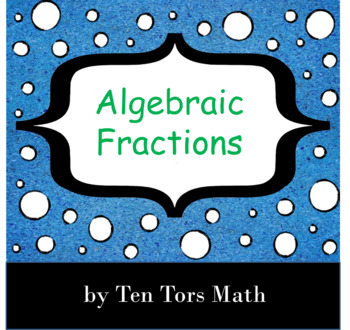# Algebraic Fractions Lesson Sequence;
8th - 12th, Homeschool
Subjects
Resource Type
Standards
Formats Included
• PPTX
Pages
44 pages
Report this resource to TPT

### Description

Algebraic Fractions

A sequence of lessons to cover 3 to 4 lessons on algebraic fractions which includes:

Simplifying Algebraic Fractions

Multiplying & Dividing Algebraic Fractions

Solving Algebraic Fraction Equations

Fully customizable PowerPoint.

If you would like to see an example of my bingo! games I have some free sample versions:

- Rational (fractional) and negative exponents here: *** FREE Rational and negative exponents Bingo! game ***

- Estimating calculations by rounding to one significant figure here: *** FREE Estimating Bingo! game ***

Total Pages
44 pages
Included
Teaching Duration
1 Week
Report this resource to TPT
Reported resources will be reviewed by our team. Report this resource to let us know if this resource violates TPT’s content guidelines.

### Standards

to see state-specific standards (only available in the US).
Interpret complicated expressions by viewing one or more of their parts as a single entity. For example, interpret 𝘗(1 + 𝘳)ⁿ as the product of 𝘗 and a factor not depending on 𝘗.
Use the structure of an expression to identify ways to rewrite it. For example, see 𝘹⁴ – 𝘺⁴ as (𝘹²)² – (𝘺²)², thus recognizing it as a difference of squares that can be factored as (𝘹² – 𝘺²)(𝘹² + 𝘺²).
Factor a quadratic expression to reveal the zeros of the function it defines.
Understand that polynomials form a system analogous to the integers, namely, they are closed under the operations of addition, subtraction, and multiplication; add, subtract, and multiply polynomials.
Understand that rational expressions form a system analogous to the rational numbers, closed under addition, subtraction, multiplication, and division by a nonzero rational expression; add, subtract, multiply, and divide rational expressions.